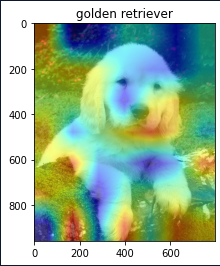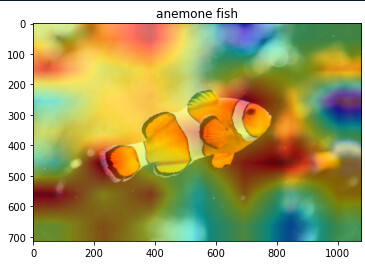# Why is my feature heatmap always incorrect?

This is the code for generating a heatmap. For some reason I have tried different images but every image heatmap looks kinda not right.
These are the heatmaps generatedAs you see in the image title the model predictions are correct, but the heatmap is not.

I wanted to ask if there is some mistake in my code, or are these the real and good heatmaps?

``````import numpy as np
from PIL import Image
import matplotlib.pyplot as plt
import torch
from torch import nn
from torch.nn import functional as F
from torchvision.models import resnet50, resnet101, resnet152, ResNet50_Weights
from torchvision.models.feature_extraction import get_graph_node_names, create_feature_extractor
import cv2

resnet50_model = resnet50(weights=ResNet50_Weights.IMAGENET1K_V2)
resnet50_model.eval()

preprocess = transforms.Compose([
transforms.Resize((224, 224)),
# transforms.CenterCrop(224),
transforms.ToTensor(),
transforms.Normalize(
mean=[0.485, 0.456, 0.406],
std=[0.229, 0.224, 0.225]
)])

image_path = './data/test_images/fish.jpeg'
image = Image.open(image_path).convert('RGB')
img_processed = preprocess(image)
batch_img_cat_tensor = torch.unsqueeze(img_processed, 0)

print(f'image shape: {image.size}, batched image shape: {batch_img_cat_tensor.shape}')

feature_extractor = create_feature_extractor(resnet50_model,
return_nodes=['layer4.2.conv3', 'fc'])

out = feature_extractor(batch_img_cat_tensor)

pred = torch.argmax(out['fc'])

last_conv_output = torch.squeeze(out['layer4.2.conv3'])
last_conv_output = torch.reshape(last_conv_output, (7, 7, -1))
last_conv_output = last_conv_output.detach().numpy() * 255.0
last_conv_output = last_conv_output.astype(np.uint8)

width_factor = int(image.size / last_conv_output.shape)
height_factor = int(image.size / last_conv_output.shape)
print(f'width factor: {width_factor}, height factor: {height_factor}')

last_conv_w, last_conv_h, n_channels = last_conv_output.shape
upscaled_h = last_conv_h * height_factor
upscaled_w = last_conv_w * width_factor

upsampled_last_conv_output = []

for x in range(0, n_channels, 512):
upsampled_last_conv_output.append( cv2.resize(last_conv_output[:, :, x:x+512], (upscaled_w, upscaled_h), cv2.INTER_CUBIC))

upsampled_last_conv_output = np.concatenate(upsampled_last_conv_output, axis=2)

last_layer_weights = resnet50_model.fc.weight.T
last_layer_weights_for_pred = last_layer_weights[:, pred]

upsampled_last_conv_output = upsampled_last_conv_output.reshape((-1, 2048))
heat_map = np.dot(upsampled_last_conv_output,
last_layer_weights_for_pred.detach().numpy()).reshape(upscaled_h, upscaled_w)

import ast

with open('./data/imagenet_labels.txt') as file: